# Time Distance and Speed Questions

Time Distance and Speed questions with answers, short tricks and solution for SSC CGL, CHSL, GD, Bank, UPSSSC PET, CUET and all Competitive Exams.

## Time Distance and Speed Questions for Competitive Exams

Question 1 : A man walking at the rate of 5 km/hr. crosses a bridge in 15 minutes. The length of the bridge (in metres) is :
(1) 600
(2) 750
(3) 1000
(4) 1250

(4) 1250 m
Speed of the man = 5km/hr
= 5 x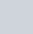$\dfrac{1000}{60}$
= \$ latex \dfrac{250}{3} \$ m / min
Time taken to cross the bridge
= 15 minutes
Length of the bridge
= speed × time
=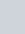$\dfrac{250}{3}$ x15 m
=1250 m

Question 2: A train is moving with the speed of 180 km/hr. Its speed (in metres per second) is :
(1) 5
(2) 40
(3) 30
(4) 50

(4) 50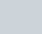$\dfrac{180x 5}{18}$ m/sec
= 50 m/sec

Question 3: A speed of 30.6 km/.hr is the same as
(1) 8.5 m/sec
(2) l0 m/sec
(3) 12 m/sec
(4) 15.5 m/sec

(1) 8.5 m/sec
(1) 30.6 kmph
= 30.6 x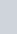$\dfrac{5}{18}$
=8.5 m/sec

Question 4: A car travels at a speed of 60 km/hr and covers a particular distance in one hour. How long will it take for another car to cover the same distance at 40 km/hr ?
(1)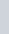$\dfrac{5}{2}$ hours
(2) 2 hours
(3)$\dfrac{3}{2}$ hours
(4) 1 hour

(3)$\dfrac{3}{2}$ hours
Distance = Speed × Time= 60 km.
Time taken at 40 kmph
=$\dfrac{60}{40}$
=$\dfrac{3}{2}$ hours

Question 5: Gautam goes to office at a speed of 12 kmph and returns home at 10 kmph. His average speed is :
(1) 11 kmph
(2) 22 kmph
(3) 10.9 kmph
(4) 12.5 kmph

(3) 10.9 kmph
Here distances are same.
Average speed =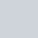$\dfrac{2xy}{x+y}$ kmph
=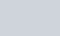$\dfrac{2x12x10}{12+10}$
=$\dfrac{240}{22}$ kmph

Question 6: A train is running at the speed of 116 km/hr. The distance covered by the train in meters in 18 seconds is:
(1) 900 metre
(2) 1160 metre
(3) 508 metre
(4) 580 metre

(4) 580 metre
Speed of train = 116 kmph
116$\dfrac{5}{18}$ m./sec
=$\dfrac{580}{18}$
Required distance = Speed × Time
=$\dfrac{580}{18}$ x 18
=580 metre

Question 7: A train 300 metres long is running at a speed of 25 metres per second. It will cross a bridge of 200 metres in
(1) 5 seconds
(2) 10 seconds
(3) 20 seconds
(4) 25 seconds

(3) 20 seconds
In crossing the bridge, the train travels its own length plus the length of the bridge.
Total distance (length)
= 300 + 200 = 500 m.
Speed = 25m/sec.
The required time
= 500 ÷ 25 = 20 seconds

Question 8: A train travelling at a speed of 30 m/sec crosses a platform, 600 metres long, in 30 seconds. The length (in metres) of train is
(1) 120
(2) 150
(3) 200
(4) 300

(4) 300
Let the length of train be x
According to the question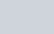$\dfrac{600+x}{30}$
x=300 m

Question 9: A train of length 500 feet crosses a platform of length 700 feet in 10 seconds. The speed of the train is
(1) 70 ft/second
(2) 85 ft/second
(3) 100 ft/second
(4) 120 ft/second

(4) 120 ft/second
Speed of train
=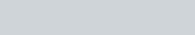$\dfrac{Length of train + platform}{Time taken to cross}$
=\$frac \dfrac{(500+700)}{10} \$
=120 ft/second

Question 10: A train 270 m long running at a speed of 36 kmph will cross a bridge 180 m long at __.
(1) 40 sec
(2) 45 sec
(3) 50 sec
(4) 35 sec

(2) 45 sec
36 kmph =36x$\dfrac{5}{18}$ =10 m/sec
Required time =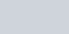$\dfrac{270+180}{10}$= 45 seconds

### Time Distance and Speed Questions for SSC CGL, CHSL

Question 11: The distance between two cities A and B is 330 km. A train starts from A at 8 a.m. and travels towards B at 60 km/hr. Another train starts from B at 9 a.m. and travels towards A at 75 km/hr. At what time do they meet?
(1) 10 am
(2) 10 : 30 am
(3) 11 am
(4) 11 : 30 am

(3) 11 am
Distance travelled by first train in one hour = 60 × 1 = 60km
Therefore, distance between two train at 9 a.m. = 330 – 60 = 270 km
Now, Relative speed of two trains = 60 + 75 = 135 km/hr
Time of meeting of two trains=$\dfrac{270}{135}$ = 2 hrs.
Therefore, both the trains will meet at 9 + 2 = 11 A.M.

Question 12: Two men are standing on opposite ends of a bridge 1200 metres long. If they walk towards each other at the rate of 5m/minute and 10m/minute respectively, in how much time will they meet each other ?
(1) 60 minutes
(2) 80 minutes
(3) 85 minutes
(4) 90 minutes

(2) 80 minutes
Men are walking in opposite directions. Hence, they will cover the length of bridge at their relative speed.
Required time=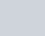$\dfrac{1200}{5+10}$ = 80 minutes

Question 13: Two towns A and B are 500 km apart. A train starts at 8 AM from A towards B at a speed of 70 km/hr. At 10 AM, another train starts from B towards A at a speed of 110 km/hr. When will the two trains meet ?
(1) 1 PM
(2) 12 Noon
(3) 12.30 PM
(4) 1.30 PM

(2) 12 Noon
Let two trains meet after t hours when the train from town A leaves at 8 AM.
Distance covered in t hours at 70 kmph + Distance covered in(t – 2) hours at 110 kmph= 500km
70t + 110 (t – 2) = 500
t= 4 hours
Hence, the trains will meet at 12 Noon.

Question 14: A train running at the speed of 84 km/hr passes a man walking in opposite direction at the speed of 6 km/hr in 4 seconds. What is the length of train (in metre) ?
(1) 150
(2) 120
(3) 100
(4) 90

(3) 100
Relative speed = (84 + 6) = 90 kmph= 25 m/sec.
Length of train= Relative speed × Time = 25 × 4 = 100 metre

Question 15: By walking at$\dfrac{3}{4}$ of his usual speed, a man reaches his office 20 minutes later than his usual time. The usual time taken by him to reach his office is
(1) 75 minutes
(2) 60 minutes
(3) 40 minutes
(4) 30 minutes

(2) 60 minutes$\dfrac{4}{3}$ of usual time = Usual time + 20 minutes$\dfrac{1}{3}$ of usual time = 20 minutes
Usual time = 20 × 3= 60 minutes

Question 16 : The speed of a car is 54 km/hr. What is its speed in m/sec?
(1) 15 m/sec
(2) 19-44 m/sec
(3) 194.4 m/sec
(4) 150 m/sec

(1) 15 m/sec
1 kmph =$\dfrac{5}{18}$ m/sec
54 kmph =$\dfrac{5}{18}$ x 54 = 15 m/sec

Question 17 : A car covers a certain distance in 25 hours. If it reduces the speed by$\dfrac{1}{5}$ th, the car covers 200 km less in that time. The speed of car is
(1) 60 km/hr
(2) 30 km/hr
(3) 40 km/hr
(4) 50 km/hr

(3) 40 km/hr
Speed of car = x kmph.
Distance = Speed × Time = 25x km.
Case II,
Speed of car =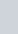$\dfrac {4x}{5}$ kmph
Distance covered =$\dfrac{4x}{5}$ x 25= 20x km
According to the question,
25x – 20x = 200
5x = 200
x =$\dfrac{200}{5}$ = 40 kmph

Question 18 : A train covers a distance of 3584 km in 2 days 8 hours. If it covers 1440 km on the first day and 1608 km on the second day, by how much does the average speed of the train for the remaining part of the journey differ from that for the entire journey ?
(1) 3 km/hour more
(2) 3 km/hour less
(3) 4 km/hour more
(4) 5 km/hour less

(1) 3 km/hour more
Remaining distance
= (3584 – 1440 – 1608) km
= 536 km.
This distance is covered at the rate of$\dfrac{536}{8}$ = 67 kmph.
Average speed of whole journey =
\$latex \dfrac{3584}{56} = 64 kmph
Required difference in speed
= (67 – 64) kmph i.e. = 3 kmph more

Question 19: A man goes from Mysore to Bangalore at a uniform speed of 40 km/hr and comes back to Mysore at a uniform speed of 60 km/hr. His average speed for the whole journey is
(1) 48 km/hr
(2) 50 km/hr
(3) 54 km/hr
(4) 55 km/hr

(1) 48 km/hr
Average speed=$\dfrac{2xy}{x+y}$
=$\dfrac {2x40x60}{40+60}$
= 48 km/hr

Question 20 : On a journey across Kolkata, a taxi averages 50 km per hour for 50% of the distance, 40 km per hour for 40% of it and 20 km per hour for the remaining. Average speed (in km/hour) for the whole journey is :
(1) 42
(2) 40
(3) 35
(4) 45

(2) 40
Total distance = 100 km.
Total time =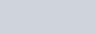$\dfrac{50}{50} +\dfrac{40}{40}+\dfrac{10}{20}$
= 1+1+$\dfrac{1}{2}$
=$\dfrac{5}{2}$
Average speed =100 x$\dfrac{2}{5}$ = 40 km/hour

Thanks for visit and attempt Time Distance and Speed Maths questions for SSC CGL, CHSL competitive exams.

Time Distance and Speed Questions in Hindi

Time, Distance and Speed Questions in Hindi

Maths Questions for Competitive Exams

Scroll to Top